Guru

# If a, b, c are in continued proportion, prove that: (a+b)/(b+c) =a2(b-c) /b2(a-b) (ii) 1/a3+1/b3+1/c3=a/b2c2+ b/c2a2+c/a2b2 (iii) a: c = (a2 + b2): (b2 + c2) (iv) a2b2c2 (a-4 + b-4 + c-4) = b-2 (a4 + b4 + c4) (v) abc (a + b + c)3 = (ab + bc + ca)3 (vi) (a + b + c) (a – b + c) = a2 + b2 + c2

• 2

exam oriented an important question from ML aggarwal, class 10th, chapter 7, ratio and proportion, Avichal publication

This question has been asked in 2015

It is given that a, b, c are in continued proportion ans we are asked to prove the equalities using the condition given in the equation.

Question 21, 7.2

Share

1. Solution:

It is given that

a, b, c are in continued proportion

So we get

a/b = b/c = k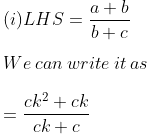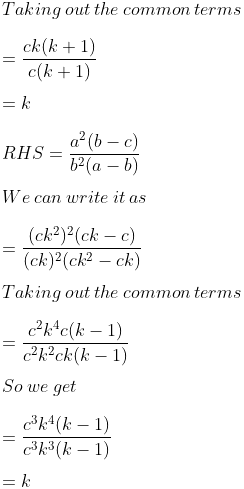Therefore, LHS = RHS.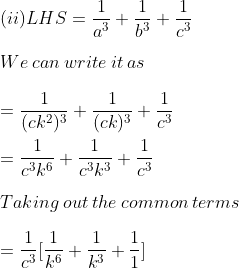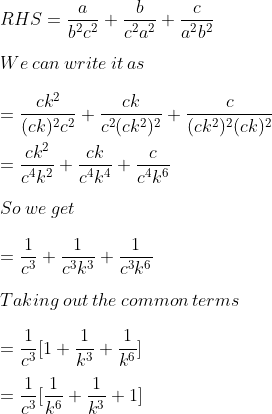Therefore, LHS = RHS.

(iii) a: c = (a2 + b2): (b2 + c2)

We can write it as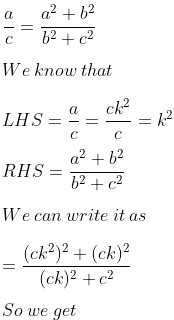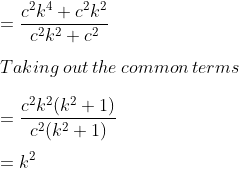Therefore, LHS = RHS.

(iv) a2b2c2 (a-4 + b-4 + c-4) = b-2 (a4 + b4 + c4)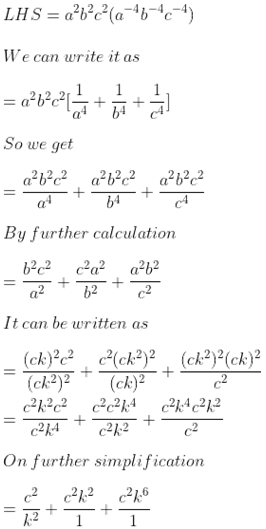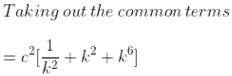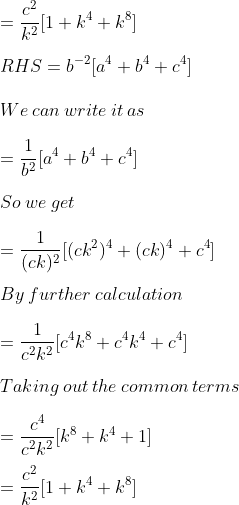Therefore, LHS = RHS.

(v) LHS = abc (a + b + c)3

We can write it as

= ck2. ck. c [ck2 + ck + c]3

Taking out the common terms

= c3 k3 [c (k2 + k + 1)]3

So we get

= c3 k3. c3 (k2 + k + 1)3

= c6 k3 (k2 + k + 1)3

RHS = (ab + bc + ca)3

We can write it as

= (ck2. ck + ck. c + c. ck2)3

So we get

= (c2k3 + c2k + c2k2)3

= (c2k3 + c2k2 + c2k)3

Taking out the common terms

= [c2k (k2 + k + 1)]3

= c6k3 (k2 + k + 1)3

Therefore, LHS = RHS.

(vi) LHS = (a + b + c) (a – b + c)

We can write it as

= (ck2 + ck + c) (ck2 – ck + c)

Taking out the common terms

= c (k2 + k + 1) c (k2 – k + 1)

= c2 (k2 + k + 1) (k2 – k + 1)

So we get

= c2 (k4 + k2 + 1)

RHS = a2 + b2 + c2

We can write it as

= (ck2)2 + (ck)2 + (c)2

So we get

= c2k4 + c2k2 + c2

Taking out the common terms

= c2 (k4 + k2 + 1)

Therefore, LHS = RHS.

• -1